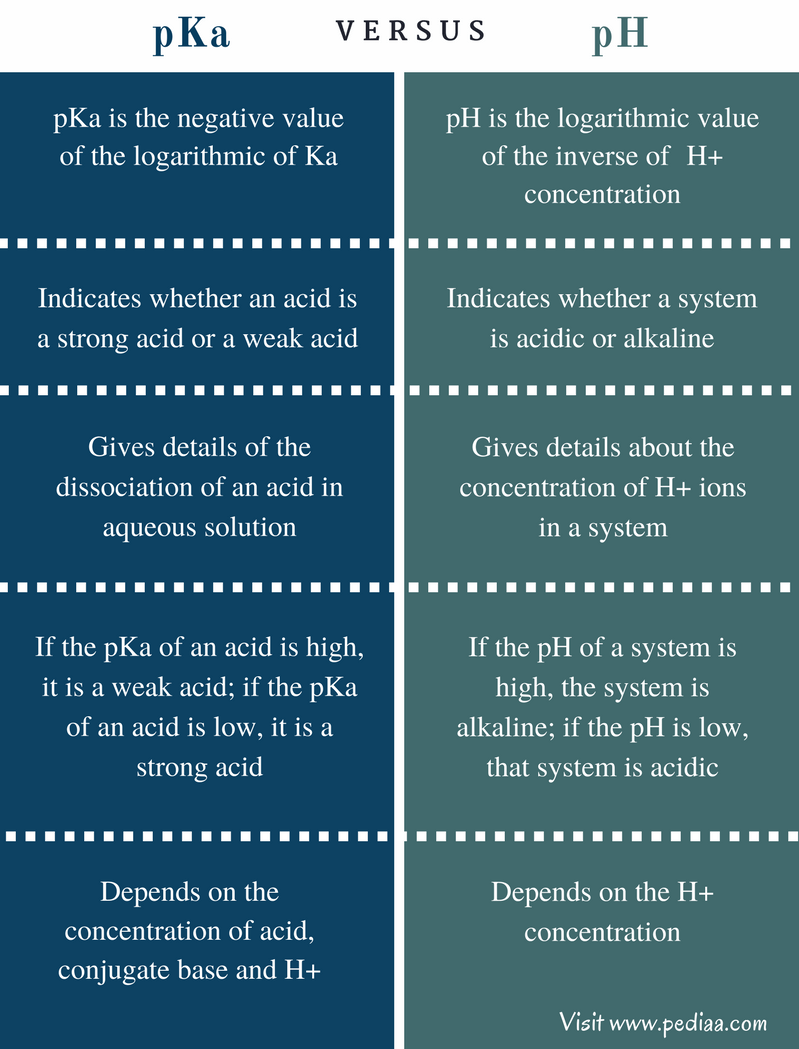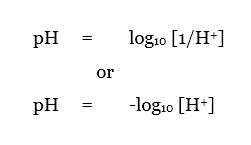# Difference Between pKa and pH

## Main Difference – pKa vs pH

In physical chemistry, pKa and pH are two terms related to the acidity of a system. These are logarithmic values. The easiest way to express and compare very large or very small amounts is to take their logarithmic value. The logarithm gives a simple value which is very easy to handle. The main difference between pKa and pH is that pKa indicates the dissociation of an acid whereas pH indicates the acidity or alkalinity of a system.

### Key Areas Covered

1. What is pKa
– Definition, Values, Relationship
2. What is pH
– Definition, Values, Relationship
3. What is the Difference Between pKa and pH
– Comparison of Key Differences

Key Terms: Acidity, Acid Dissociation Constant, Basicity, pH, pKa## What is pKa

pKa is the negative value of the logarithm of Ka. Ka is the symbol given for acid dissociation constant. Some acids are strong acids while some are weak acids. Strong acids completely dissociate into its ions when it is in aqueous solutions. But weak acids partially dissociate, generating an equilibrium between the acid and its conjugated base. For example, if we consider a weak acid named HA, its dissociation can be given as below.The acid dissociation constant of this equilibrium is,

Ka       =       [A][H+]/[HA]

Where,

[A]   = Concentration of the conjugate base

[H+]  = Concentration of H+ ions

[HA] = Concentration of Acid present

Then the pKa value of the acid dissociation constant can be indicated as below.

pKa   =   – log10[Ka]

By looking at the pKa value, we can determine whether an acid is a strong acid or a weak acid. If the value of pKa is high, the acid is weak. This is because a higher pKa value indicates that Ka is low. In order for the value of Ka to be low, [A][H+] value should be lower than the value of [HA]. This means that the acid has been dissociated partially. But if the value of [A][H+] is higher than [HA], then the Ka would be high, and pKa would be low correspondingly. This indicates that it is a strong acid.

## What is pH

pH is the logarithmic value of the inverse of  H+ concentration. pH value indicates the acidity or alkalinity of a system. If the pH value of a particular system is high, then it is called an alkaline or basic system. If the pH value of a system is low, that system is acidic. The range of pH is from 1 to 14. The pH value 7 is known to be the neutral pH where no acidity or alkalinity is present. The relationship between pH and pKa can be shown as below.In analytical chemistry, pH indicators are used to identify the endpoint of a certain reaction. These indicators are very sensitive to pH changes, and they show different colors for different pH values in aqueous solutions.

However, pH value is a good indication of the acidity or alkalinity in a water body. pH meters can be used to measure the pH of water or any other solution.

## Difference Between pKa and pH

### Definition

pKa: pKa is the negative value of the logarithmic of Ka.

pH: pH is the logarithmic value of the inverse of  H+ concentration.

### Indication of Acidity

pKa: pKa indicates whether an acid is a strong acid or a weak acid.

pH: pH indicates whether a system is acidic or alkaline.

### Details Given

pKa: pKa gives details of the dissociation of an acid in aqueous solution.

pH: pH gives details about the concentration of H+ ions in a system.

### Values

pKa: If the pKa of an acid is high, it is a weak acid, and if the pKa of an acid is low, it is a strong acid.

pH: If the pH of a system is high, the system is alkaline, but if the pH is low, that system is acidic.

### Effect of Other Parameters

pKa: pKa is dependent on the concentration of acid, conjugate base and H+.

pH: pH depends on the H+ concentration.

### Conclusion

Both pKa and pH are very important parameters in laboratory practices. The main difference between pKa and pH is that pKa indicates the dissociation of an acid whereas pH indicates the acidity or alkalinity of a system.

##### References:

1.”PH.” What is pH. N.p., n.d. Web. Available here. 04 July 2017.
2.”PKa and Dissociation Equilibrium.” PKa and Dissociation Equilibrium : SHIMADZU (Shimadzu Corporation). N.p., n.d. Web. Available here. 04 July 2017.